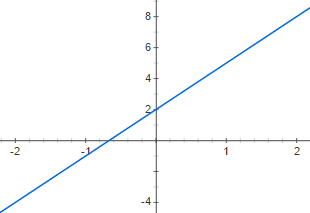# Linear Equations in College Algebra Flashcards

Linear Equations in College Algebra Flashcards
1 / 14 0 0
14 cards in set

## Flashcard Content Overview

This flashcard set will help you understand linear equations including slope, x- and y-intercepts, and systems of equations. Use this set to test your linear equation knowledge and skills to prep you for questions you may encounter on your College Algebra exam.

Front
Back
Two points on a line are (2,2) and (3,1). Find the slope of the line.
The slope of the line is -1 (m = -1)
Parallel lines always/never have the same slope
Parallel lines always have the same slope
Two points on a line are (2,2) and (3,1). Find the y-intercept of the line.
The y intercept is (0,4)
The line y = -x + 4 will/will not enter the third quadrant
The line y = -x + 4 will not enter the third quadrant
In the equation y = mx + b, m stands for _____ and b stands for _____
m = slope and b = y-intercept
The three main ways to solve a system of equations are _____, _____ , and _____ .
Graphing, substitution, and elimination are the three main ways to solve a system of equations
Solve this system of equations
2x + y = 4
x + 2y = 2
(2,0)
Find the slope and y-intercept of the following equation: 2y = 4x + 5
m = 2
y-intercept = (0,5/2)
The line y = 3/4x + 5 will be parallel/perpendicular to the line y = -4/3x -3
These lines will be perpendicular
The equation for the line shown below is _____y = 3x+2
The x and y intercepts of 4x + 2y = 8 are _____
x-intercept = (2,0) and y-intercept = (0,4)
If a line is completely vertical, that line has a(n) undefined/zero slope.
If a line is completely vertical, that line has an undefined slope
If a line is completely horizontal, that line has a(n) undefined/zero slope.
If a line is completely horizontal, that line has a zero slope
The slope of the line shown below is _____The slope of the line is 3 (m=3)

To unlock this flashcard set you must be a Study.com Member.